#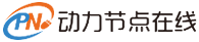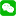微信登录微信登录与注册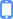手机号登录

# 数据结构堆的实现

08-03 10:53 568浏览

• 大字
• 中字
• 小字

## 符合以下规则：

1.元素可比较性：数据集中的元素可以进行比较，就是要实现Comparable接口;。

2.节点最大/最小性：每个节点的元素必须大于或小于该节点的孩子节点的元素;

3.堆是一棵完全二叉树。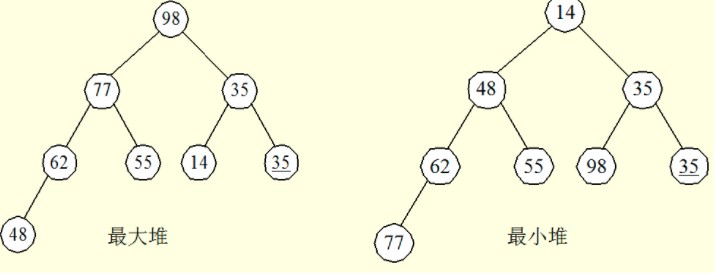## 堆的大小是提前知道的，在java集合中堆是通过ArrayList数组实现的：

1.根节点位置：根节点的数据总是在数组的位置

2.节点的父节点位置：假设一个非根节点的数据在数组中的位置[i]，那么它的父节点总是在位置[(i-1)/2]

3.节点的孩子节点位置：假设一个节点的数据在数组中的位置为[i]，那么它的孩子(如果有)总是在下面的这两个位置：左孩子在[2*i+1]，右孩子在[2*i+2]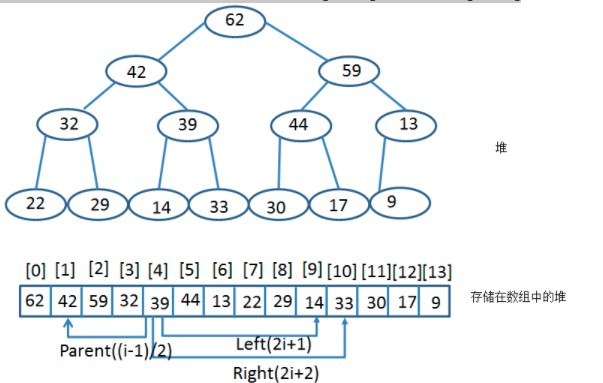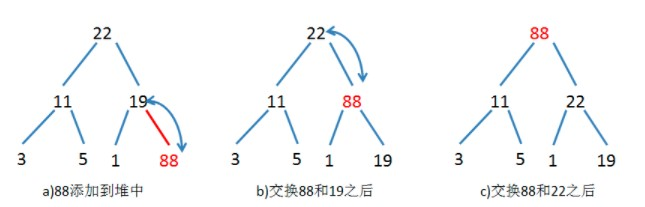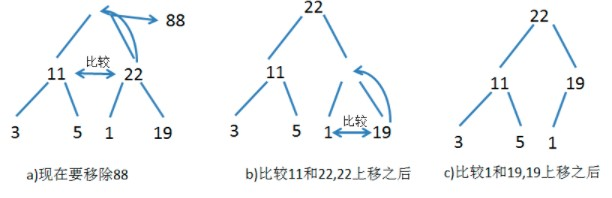heap.java实现

``````//最大堆
import java.util.ArrayList;
public class Heap<E extends Comparable>{
private ArrayList<E> list=new ArrayList<E>();//用数组实现堆
public Heap(){}
public Heap(E[] objects){
for(int i=0;i<objects.length;i++){
}
}
int currentIndex=list.size()-1;
while(currentIndex>0){
int parentIndex=(currentIndex-1)/2;//找到该结点的父结点
if(list.get(currentIndex).compareTo(list.get(parentIndex))>0){//与父节点比较
//如果当前结点的值大于父结点就交换位置
E temp=list.get(currentIndex);
list.set(currentIndex, list.get(parentIndex));
list.set(parentIndex, temp);
}
else
break;
currentIndex=parentIndex;
}
}
public E remove(){//删除并返回根结点,堆的特点是移除了根结点后还是堆
if(list.size()==0) return null;
E removeObject=list.get(0);
list.set(0, list.get(list.size()-1));//把最后一个结点放在根结点的位置
list.remove(list.size()-1);
int currentIndex=0;
while(currentIndex<list.size()){
int leftChildIndex=2*currentIndex+1;
int rightChildIndex=2*currentIndex+2;//左右孩子结点的坐标
if(leftChildIndex>=list.size())break;
//比较左右孩子的值，使maxIndex指向值大的结点
int maxIndex=leftChildIndex;
if(rightChildIndex<list.size()){
if(list.get(maxIndex).compareTo(list.get(rightChildIndex))<0){
maxIndex=rightChildIndex;
}
}
//如果当前结点的值小于其左右孩子中的大的值，就交换两个结点
if(list.get(currentIndex).compareTo(list.get(maxIndex))<0){
E temp=list.get(maxIndex);
list.set(maxIndex, list.get(currentIndex));
list.set(currentIndex, temp);
currentIndex=maxIndex;
}
else
break;
}
return removeObject;

}
public int getSize(){
return list.size();
}
}``````

0人推荐

0条评论

Java面试题及答案整理

Mybatis返回值类型

Java string类详解

6道经典算法面试题

## 举报

0/150